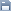Leetcode
2020.04.25 06:41

# 443. String Compression

조회 수 103 추천 수 0 댓글 0
?

#### 단축키

Prev이전 문서

Next다음 문서

크게 작게 위로 아래로 댓글로 가기 인쇄
?

#### 단축키

Prev이전 문서

Next다음 문서

크게 작게 위로 아래로 댓글로 가기 인쇄

Given an array of characters, compress it in-place.

The length after compression must always be smaller than or equal to the original array.

Every element of the array should be a character (not int) of length 1.

After you are done modifying the input array in-place, return the new length of the array.

Follow up:
Could you solve it using only O(1) extra space?

Example 1:

```Input:
["a","a","b","b","c","c","c"]

Output:
Return 6, and the first 6 characters of the input array should be: ["a","2","b","2","c","3"]

Explanation:
"aa" is replaced by "a2". "bb" is replaced by "b2". "ccc" is replaced by "c3".
```

Example 2:

```Input:
["a"]

Output:
Return 1, and the first 1 characters of the input array should be: ["a"]

Explanation:
Nothing is replaced.
```

Example 3:

```Input:
["a","b","b","b","b","b","b","b","b","b","b","b","b"]

Output:
Return 4, and the first 4 characters of the input array should be: ["a","b","1","2"].

Explanation:
Since the character "a" does not repeat, it is not compressed. "bbbbbbbbbbbb" is replaced by "b12".
Notice each digit has it's own entry in the array.
```

Note:

1. All characters have an ASCII value in `[35, 126]`.
2. `1 <= len(chars) <= 1000`.

```class Solution {
public int compress(char[] chars) {
List<Character> list = new ArrayList<>();
char curr = chars;
int count = 1;

for(int i=0; i<chars.length; i++){
int next = i + 1;
if(next < chars.length && chars[i] == chars[next]){
count++;
}else{
list.add(curr);
curr = chars[next<chars.length?next:i];
if(count > 1){
for(int j=0; j<(count+"").length(); j++){
list.add((count+"").charAt(j));
}
}
count = 1;
}
}

for(int i=0; i<list.size(); i++){
//System.out.println(list.get(i));
chars[i] = list.get(i);
}

return list.size();
}
}```

```class Solution {
public int compress(char[] chars) {
List<Character> list = new ArrayList<>();
int curr = 0;
int count = 1;

for(int i=0; i<chars.length; i++){
int next = i + 1;
if(next < chars.length && chars[i] == chars[next]){
count++;
}else{
list.add(chars[curr]);
curr = next < chars.length ? next : i;
if(count > 1){
for(int j=0; j<(count+"").length(); j++){
list.add((count+"").charAt(j));
}
}
count = 1;
}
}

for(int i=0; i<list.size(); i++){
//System.out.println(list.get(i));
chars[i] = list.get(i);
}

return list.size();
}
}```

```class Solution {
public int compress(char[] chars) {
int index = 0;
int buffer = 0;

for (int i = 0; i < chars.length; i++) {
int next = i + 1;
if (next == chars.length || chars[i] != chars[next]) {
chars[buffer++] = chars[index];
if (index < i) {
String count = (i - index + 1) + "";
for(int j=0; j<count.length(); j++){
chars[buffer++] = count.charAt(j);
}
}
index = next;
}
}
return buffer;
}
}```

?

 제목+내용제목내용댓글이름닉네임아이디태그
List of Articles
번호 분류 제목 글쓴이 날짜 조회 수
74 Leetcode 692. Top K Frequent Words 2020.04.15 87
73 Leetcode 2. Add Two Numbers 2020.04.14 90
72 Leetcode 731. My Calendar II 2020.04.15 94
71 Leetcode 75. Sort Colors 2020.04.14 95
70 Leetcode 347. Top K Frequent Elements 2020.04.14 96
69 Leetcode 430. Flatten a Multilevel Doubly Linked List2020.04.06 98
68 Leetcode 23. Merge k Sorted Lists 2020.04.14 98
67 Leetcode 729. My Calendar I 2020.04.15 98
66 Leetcode 253. Meeting Rooms II 2020.04.15 98
65 Leetcode 20. Valid Parentheses 2020.04.25 98
64 Leetcode 62. Unique Paths2020.04.09 99
63 Leetcode 7. Reverse Integer 2020.04.28 100
62 Leetcode 946. Validate Stack Sequences 2020.04.08 101
61 Leetcode 973. K Closest Points to Origin 2020.04.15 101
60 Leetcode 852. Peak Index in a Mountain Array 2020.04.28 101
59 Leetcode 350. Intersection of Two Arrays II 2020.04.28 102
» Leetcode 443. String Compression 2020.04.25 103
57 Leetcode 38. Count and Say 2020.04.25 103
56 Leetcode 53. Maximum Subarray 2020.04.28 106
55 Leetcode 3. Longest Substring Without Repeating Characters 2020.04.09 107
Board Pagination Prev 1 2 3 4 Next
/ 4